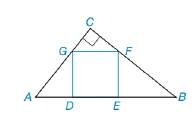Chapter 8.1, Problem 53EElementary Geometry For College St...

7th Edition
Alexander + 2 others
ISBN: 9781337614085

Solutions

Chapter
SectionElementary Geometry For College St...

7th Edition
Alexander + 2 others
ISBN: 9781337614085
Textbook Problem

Square DEFG is inscribed in right △ A B C , as shown. If A D = 6 and E B = 8 , find the area of square DEFG.To determine

To find:

The area of the square DEFG.

Explanation

Finding the area of the square is followed by the geometric formula,

Area of the square A=s2 unit2,

Where s is the length of the each side.

Calculation:

Given:

Square DEFG is inscribed in right ABC, as shown below.

If AD=6 and EB=8

Let x be the length of each side of the equare.

By the triangle ABC,

Let x be the angle of LB and θ be the angle of LA.

(i.e.) LA=LF and LB=LG

By the figure,

tanθ=8x=x6

(i

Still sussing out bartleby?

Check out a sample textbook solution.

See a sample solution

The Solution to Your Study Problems

Bartleby provides explanations to thousands of textbook problems written by our experts, many with advanced degrees!

Get Started

Expand each expression in Exercises 122. (x+yxy)2

Finite Mathematics and Applied Calculus (MindTap Course List)

Define sampling with replacement and explain why is it used?

Statistics for The Behavioral Sciences (MindTap Course List)

If limx0sinxx=1, the angle x must be measured in: a) radians b) degrees c) it does not matter

Study Guide for Stewart's Single Variable Calculus: Early Transcendentals, 8th